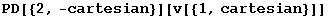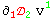7.5. Components and derivatives

ComponentValue also works with derivatives of tensors (but not for more general expressions, you can use Coba as a placeholder and then substitute the expression). Remember that with our definitions the components of the derivative are not the derivatives of the components.

In:=Out=In:=Out=In:=Out=In:=Out=Derivatives are represented by  additional central arguments in ValID:

In:=Out=

 ValID[v,PDpolar[-cartesian],PD[-polar],{{cartesian,-cartesian,-polar}}] ValID[v,PD[-cartesian],{{cartesian,-cartesian}}]

In:=Created by Mathematica  (May 16, 2008)